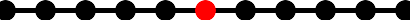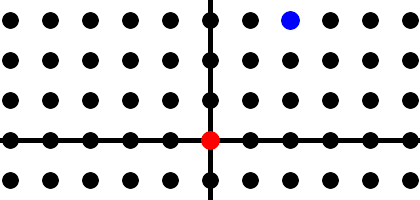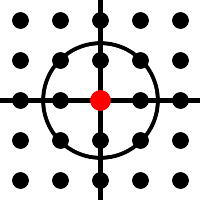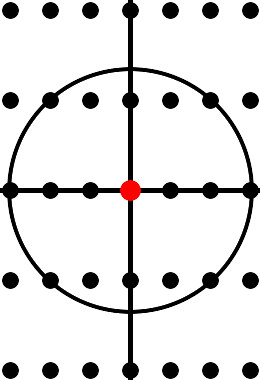# The Beauty of Prime Numbers

Let's take a break from science, and dabble in pure mathematics. Well … it was pure, until 1977, when it became applied. Now it is at the heart of internet security. It keeps your credit card numbers safe from prying eyes as you order your merchandise online, and it keeps your banking information safe as you review your recent transactions from your smart phone.

Rewind to 300 B.C., when Euclid wrote The Elements, a compendium of all mathematics known at the time. He developed geometry from 5 basic axioms, still called Euclidean geometry today, and he explored the principles of arithmetic, including prime numbers. A prime is a number that is divisible by only 1 and itself. (For technical reasons, 1 is excluded from this list.) 11 is prime because only 1 and 11 divide evenly into 11. 9 is not prime because 9 = 3 times 3. 35 is not prime, being 5 times 7, but 37 is prime. Take a moment and verify these are the first few primes: 2, 3, 5, 7, 11, 13, 17, 19, 23, 29, 31, 37. 2 is the only even prime, as every other even number is divisible by 2. 2 is also the smallest prime.

Two thousand years ago, primes had no practical application; they didn't help you build a temple, or predict the motion of the stars, or maintain an accounting of your livestock. But the Greeks were interested in math for its own sake, for the sheer beauty of the subject. Euclid investigated prime numbers, and made some important discoveries and conjectures.

One of Euclid's first theorems states there are an infinite number of primes. The primes continue forever. It's a beautiful proof, and not obvious - well - not until you see it - then it's obvious. Pause and see if you can prove it.

Suppose there are a finite number of primes. Write them down in a list, then multiply them together and call the result n. n is now divisible by every prime. It is even, and divisible by 3, and 5, and 7, and so on. n+1 is not divisible by any of these primes. The remainder is always 1. If n+1 is prime then it is a new prime. If it is not prime it is divisible by x, and x could be prime or it could be divisible by a smaller number y, and y could be prime but if it is not it is divisible by a smaller number z, and so on. Eventually, p is some prime that divides n+1. p is not on our list and is a new prime. There you have it - there are an infinite number of primes. There is no largest prime; there will always be a bigger one. How beautiful is that‽

Euclid knew, at an intuitive level, that every number factors uniquely into a product of primes. 60 is 2 times 2 times 3 times 5, and it can't be anything else. It isn't also 7 times 11. A number could be huge, with a million digits, still it factors uniquely into a product of primes. He couldn't prove it however, because the proof is not easy. The proof had to wait for the mind of Gauss, 2,000 years later. I'm not going to present the proof here, it is beyond the scope of this book, but I would like to recount a story from Gauss' childhood that illustrates his awesome genius, and awesome is not an exaggeration. One of my professors at Berkeley remarked, “Reading his Disquisitiones Arithmeticae, that's reason enough to learn German.”

When Gauss was ten years old, his teacher gave his talkative and fidgety class an assignment that was sure to keep them busy for quite a while. The students were told to add up the numbers from 1 to 100. Pause and see if you can find a way to do this quickly, without a calculator, as there was no such thing in 1787. Remember, Gauss solved this problem at age ten.

Gauss grouped the integers into pairs: 1+100, 2+99, 3+98, 4+97, etc, and there were 50 such pairs, each pair contributing 101, thus the answer is 5050. He placed his slate on the teacher's desk, sat back down, and waited for his classmates to finish their laborious calculations. (In some versions of the story, a different arithmetic progression is assigned, but the idea is the same.) A child prodigy, Gauss had discovered the formula for the sum of the first n integers, namely n×(n+1)/2. His teacher promptly referred him to a private tutor, where is gift could be nurtured. As an adult, he would prove unique factorization, and many other theorems touching almost every branch of mathematics. If you've taken multivariable calculus, you will recognize the divergence theorem, also called Gauss' theorem.

## Irrational Numbers

The Greeks drew number lines, just as we do today. They even knew about negative numbers. After all, you might owe your neighbor 3 sheep, whence you have negative 3 sheep. If somebody gives you 3 sheep, then you have to give them to your neighbor, leaving you none; thus -3 + 3 = 0. Integers on the number line are denoted by dots or circles, with the origin at the center, positive numbers to the right, and negative numbers to the left. In this illustration, the origin is red.Fractions pack the number line. Between every two fractions is another fraction, and so on. These were called ratios, or rational numbers. Between 0 and 1 we have 1/2, 3/4, 2/5, 3/7, and so on. Euclid wondered if there were numbers on the number line that aren't fractions. Is there a number that is not a ratio, i.e. not a rational number? There are, and today we call them irrational numbers, not rational. Euclid's proof is simple and beautiful. However, it assumes something that he couldn't prove, it assumes every number is uniquely a product of primes. Let's say that is true and proceed.

Euclid was familiar with the Pythagorean theorem, as described in chapter 1. If a right triangle has legs of length 1, its hypotenuse, its longest side, has length sqrt(2). Thus we can construct a distance of sqrt(2) in the plane. This distance is a point on the numberline somewhere between 1 and 2. It's not quite 3/2, and it's a little more than 7/5. You can approach it with fractions to arbitrary precision, but is it exactly equal to a fraction? Euclid said no.

Let q be the square root of 2, and suppose q is the fraction a/b. Assume a/b is in lowest terms. If a and b are both divisible by 7 then divide 7 out of both of them and find the same ratio. Thus a and b have no primes in common. Remember, the square of a/b is 2.

a2/b2 = 2

a2 = 2b2

The right side is even so the left side is even. That means a is even. The left side, a2, is now divisible by 4. The same primes appear on the left and the right. If 2×2 is part of the factorization of the left, it is part of the factorization of the right. That means 2 divides b, and b is even. Both a and b are even, and that is a contradiction. Therefore there are points along the number line that aren't rational, an entire continuum of points that we can hardly imagine.

## Gaussian Integers

If you aren't familiar with complex numbers and the complex plane, you can skip ahead to factorization below.

As you know, the square of a positive number is positive, and the square of a negative number is also positive. There is no square root of -1, so we simply invent one, and call it i, for imaginary. i2 = -1, because I said so.

The number line now expands into a number plane, also called the complex plane. The real numbers run along the horizontal axis, which is analogous to the x axis, and the imaginary numbers run along the vertical axis, which is analogous to the y axis. Once again we can denote the points with integer coordinates by dots or circles. Gauss did just that, and they are called Gaussian integers today. To find 2+3i, start at the origin, move 2 units to the right, 2 steps along the traditional number line, then move 3 steps up. How far are you from the origin? Remember the pythagorean theorem. One leg is 2 and the other is 3, and the distance to the origin is the hypotenuse, thus sqrt(22+32), or sqrt(13), or 3.605. In this plane, the origin is red, and 2+3i is blue.How do we know that 13 is a prime number? Because nothing smaller goes into it. We don't have to ask if 79 goes into 13; it's too big. We only need test 2 through 12. Actually we only need test 2 through 4, because 5 times 5 is 25, so if 13 is x times y, then either x or y is less than 5. We only need test divisors up to the square root of n. A similar result holds in the complex plane. If x and y are two points in the complex plane, and z = x times y, then the distance from z to the origin is the distance to x times the distance to y. Try this with 3+4i times 5+12i. The distance from 3+4i to the origin is the square root of 32 + 42, or the square root of 25, or 5. (Yes, these numbers are chosen to make things come out even.) The distance from 5+12i to the origin is the square root of 52 + 122, or the square root of 169, or 13. If all goes well, the product will be 65 units away from the origin.

3+4i times 5+12i =

(3+4i)×5 + (3+4i)×12i =

15 + 20i + 36i + 48i2 =

15 + 56i - 48 =

-33 + 56i

33 squared is 1089, and 56 squared is 3136. Their sum is 4225, and sqrt(4225) = 65, so we're good.

Multiplication of distances is Demoivre's Formula, and it's not hard to prove, but it's just tedious algebra, so I'll leave it to you.

Returning to the gaussian integers, 1+i is prime, because nothing smaller than 1+i divides into it. By smaller, we mean closer to the origin. Here's another picture of the complex plane with a circle passing through 1+i. The only points inside the circle are 1, i, -1, and -i. These are all forms of 1. You could divide 13 by -1 and get -13, but that's just another form of 13. Divide by -1 again to get 13 back again. Or you could divide it by i, or -i. So these aren't really factors. 1+i has no proper factors, and is prime.In the gaussian integers, 1+i is prime, but 2 is not; because 2 = 1+i times 1-i. Similarly, 17 is no longer prime, because 17 is 4+i times 4-i. The primes are different in this world, but still, every gaussian integer is uniquely a product of primes. Gauss proved this, using essentially the same proof he used for the whole numbers.

All this is leading up to a ring where unique factorization fails, i.e. there is some n that is a product of primes in two different ways, so hang on to your hat. It is somewhat counterintuitive, but math is full of unusual structures.

The gaussian integers build a pattern of squares in the plane, like a checkerboard, but what if we spread the points apart in the vertical direction, so they form rectangles, rather than squares. Let q be the square root of -5. This is a point on the imaginary axis, 2.236 units above the origin. Let multiples of q run up the imaginary axis, just as the integers run along the real axis. We are interested in the integers plus the integer multiples of q. 3+4q, 2-7q, -6+39q, etc. Here is such a plane, with the origin in red, and a circle of radius 3.What is the distance from 3 to the origin? Well 3 of course. How about 2+q? This is a right triangle whose legs have lengths 2 and sqrt(5). Apply the pythagorean theorem and the result is the square root of 4+5, or sqrt(9), or 3. The circle of radius 3 passes through the point 3, and the point 2+q.

Is 3 prime? There are only a few points inside this circle, only a few points closer to the origin. 2 is closer, but 2 does not go into 3. The result is 3/2, which is not one of the points on our grid. Similarly, 2 does not go into 2+q; the result would be 1+½q, which is not on our grid. 3 and 2+q are both prime.

Now consider the number 9. It is 3 times 3, but it is also 2+q times 2-q. Expand the latter and get 2×2 + 2q - 2q - q2, and since q2 = -5, this comes out 9. 9 factors into primes in two different ways. With this example in hand, it is not clear that factorization of the integers is unique; but it is, and Gauss proved it, using a method that still amazes us today.

## Factorization

Factoring a number into its primes is difficult, even for the fastest computers. If the number has 10 or 20 digits it's not hard, but if the number has 300 digits, it could take years. (On a unix based machine, type: factor 7382937281, and get 41 × 271 × 664471.) In 1977, a team of three mathematicians, Ron Rivest, Adi Shamir, and Len Adleman, discovered that factorization could be used to build an encryption scheme. If n is the product of two very large primes, a message, such as your credit card number, can be scrambled using the number n, and it can only be unscrambled by using the prime factors of n. An eavesdropper cannot unscramble the message, because he cannot factor n, even with powerful computers. This has become the heart of Internet security. When you check your bank balance, your computer is using a very large number n to encrypt the message, and only the bank can decrypt it, because only the bank knows the factors of n. I'm glossing over some details, but that is the idea. Euclid knew primes were beautiful in 300 B.C., today we know they have a vital role to play in our economy and in global security.